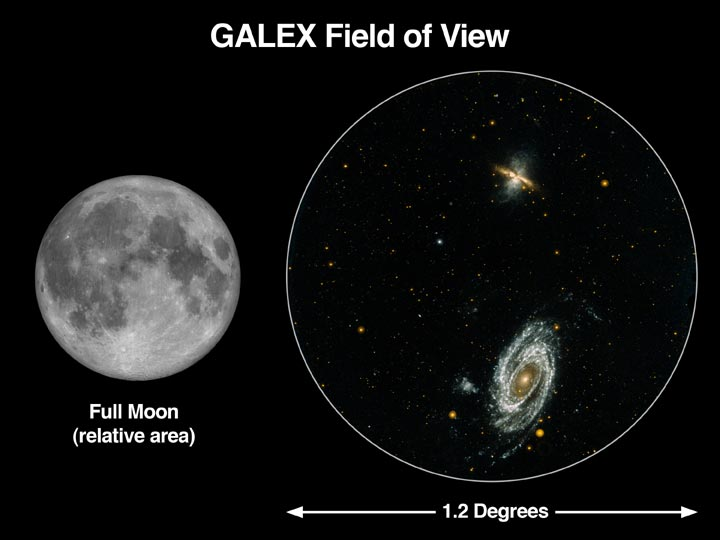In astronomy, field of view (FOV) is the amount of sky seen by the naked eye or through a telescope. This commonly refers to the angular area viewed by a telescope or any high magnification instrument; and is usually measured in square degrees, or for higher magnification instruments, in square arc-minutes.

Separated from a telescope, a typical eyepiece would have a FOV of 40? to 65? and above; this is regarded as the apparent field of view. A smaller, true or actual field of view results when the eyepiece is inserted into the telescope. To calculate the actual field of view of a telescope, divide the apparent field

of view with the telescope?s magnification. For example, a telescope with a magnification of x40 will have a 1? FOV if the eyepiece used in it has an apparent FOV of 40?.

If you don?t know your telescope?s magnification or the FOV of its eyepiece, you can easily calculate the field of view of any telescope combined with any eyepiece using the drift method. In this method, you point the telescope at a star near the celestial equator and time how long that star moves across the telescope?s field of view (from edge to edge). This can be calculated using the following formula:

FOV = (Drift time) x cosine (star?s declination) x 360? / 86,164 seconds

Or with a much simpler formula:

FOV = drift time/240 seconds